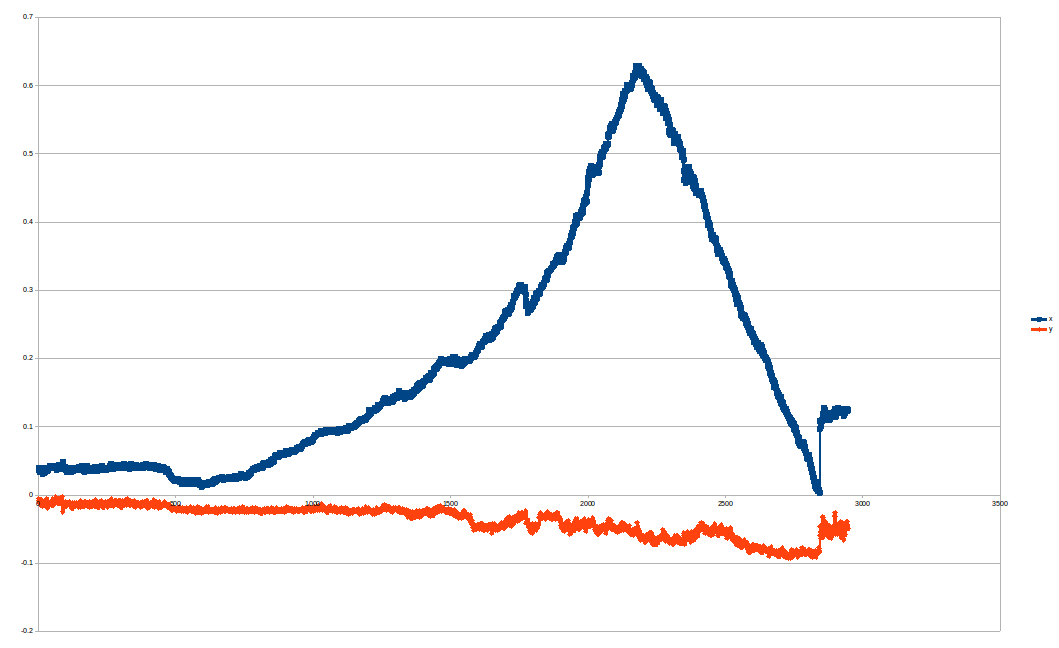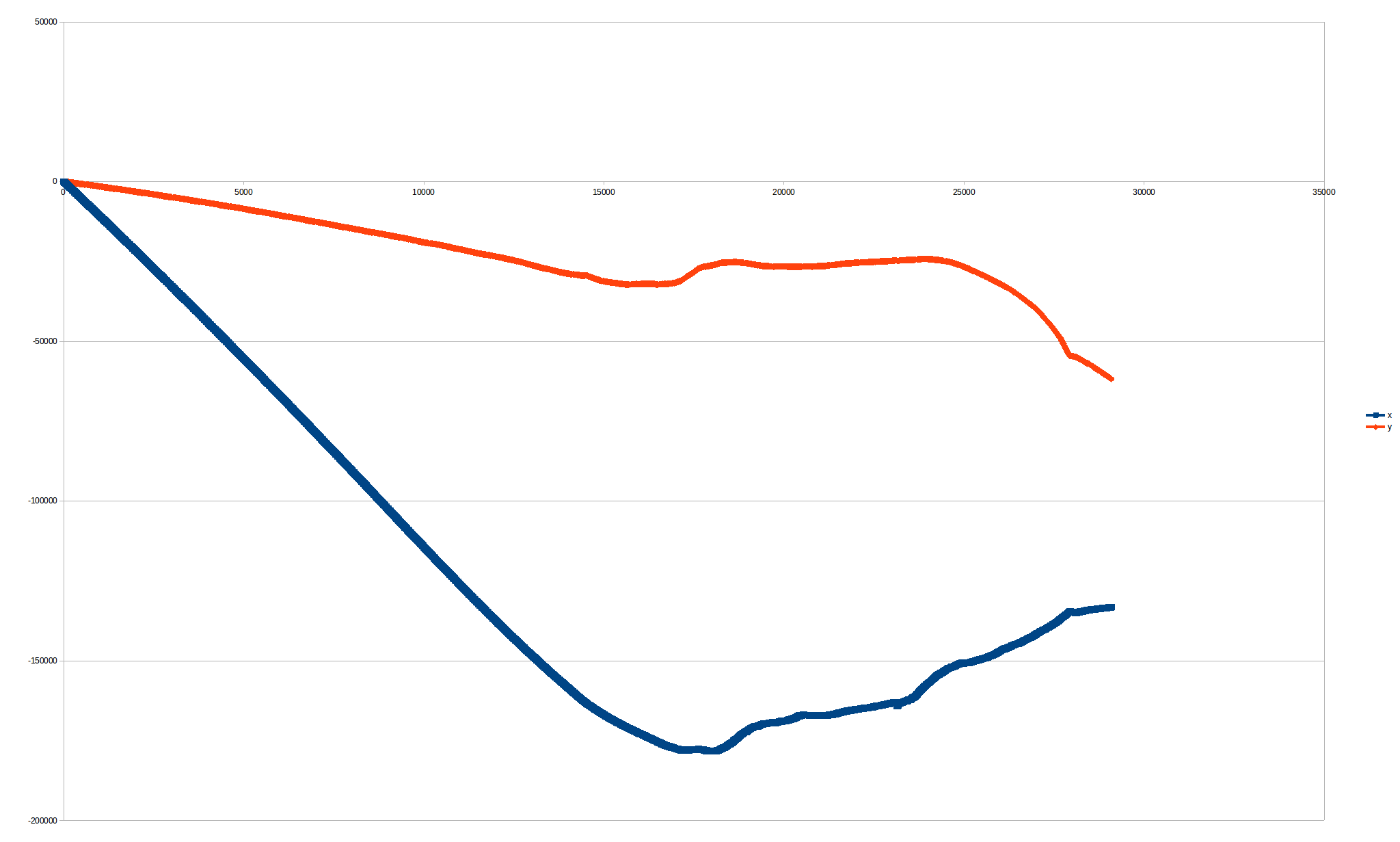# Sensor fusion (ethzasl_msf) not working well

My quadcopter starts in a place and stays there for 20 seconds, approximately. Then, it moves to the left 10 or 20 cm and then it goes back to the starter point. When I plot that using the SVO package, it gives a successful output, but with wrong units:but when I fusion that with the IMU, this is what I get:I tried changing the noise levels of the IMU, but it works even worse.

This is the pose_sensor.launch file:

<launch>
<node  launch-prefix="gdb -ex run --args" name="msf_pose_sensor" pkg="msf_updates" type="pose_sensor" clear_params="true" output="screen">
<!--remap from="msf_core/imu_state_input" to="/imu0" /-->
<remap from="msf_core/imu_state_input" to="/ardrone/imu" />
<!--remap from="msf_core/imu_state_input" to="/auk/fcu/imu" /-->
<!--remap from="msf_core/imu_state_input" to="/mav1/fcu/imu" /-->

<!--remap from="msf_updates/pose_with_covariance_input" to="/ar_pose_marker" /-->
<!--remap from="msf_updates/pose_with_covariance_input" to="/auk/down/vslam/pose" /-->
<remap from="msf_updates/pose_with_covariance_input" to="/svo/pose" />

</node>
</launch>


and this is the pose_sensor_fix.yaml (I'm plotting a rosbag):

core/data_playback: true            # Set to true for playback, set to false on the real system.

##############################
#########IMU PARAMETERS#######
##############################
# The IMU measurement model used in msf contains two types of sensor errors,
# a high frequency additive white noise and
# a slower varying sensor bias.
# https://github.com/ethz-asl/kalibr/wiki/IMU-Noise-Model-and-Intrinsics
#
# The white noise is characterized with the continuous time noise spectral density.
# The noise spectral density is sometime also referred to as noise density.
# The units of the noise spectral density are:
#  acc:  [m/s^2/sqrt(Hz)]
# The noise spectral density can be found in the datasheet of the IMU.
#
# The variation of the bias is characterized as a random walk.
# The units of the random walk are:
#  acc:  [m/s^3/sqrt(Hz)]

#core/core_noise_acc: 0.0022563    # [m/s^2/sqrt(Hz)]
#core/core_noise_gyr: 0.0004       # [rad/s/sqrt(Hz)]

#agrando ruidos (<(0.05;0.005))
core/core_noise_acc: 0.01
core/core_noise_gyr: 0.001

#core/core_fixed_bias: false
#core/core_noise_accbias: 8e-5     # [m/s^3/sqrt(Hz)]
#core/core_noise_gyrbias: 3e-6     # [rad/s^2/sqrt(Hz)]

####### mpu6000
#core/core_noise_acc: 0.003924    # [m/s^2/sqrt(Hz)] mpu6000 datasheet
#core/core_noise_gyr: 0.00008726  # [rad/s/sqrt(Hz)] mpu6000 datasheet

core/core_fixed_bias: true
core/core_noise_gyrbias: 0.0     # For fixed bias we do not need process noise.
core/core_noise_accbias: 0.0     # For fixed bias we do not need process noise.

#######################################
#########Pose Sensor Parameters #######
#######################################
pose_sensor/pose_absolute_measurements: true
pose_sensor/pose_measurement_world_sensor: false  # Selects if sensor measures its position w.r.t. world (true, e.g. Vicon) or the position of the world coordinate system w.r.t. the sensor (false, e.g. ethzasl_ptam).
pose_sensor/pose_delay: 0.02                      # [s] delay of pose sensor w.r.t. imu

# For the pose sensor noise ...
edit retag close merge delete